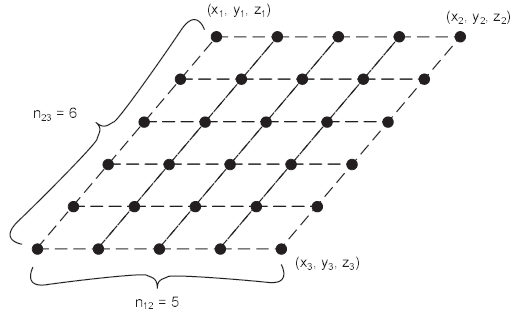# block thermal command

Syntax

block thermal keyword <range>

Primary keywords:

Specify configuration for thermal calculations. All commands shown here are only applicable if the Thermal Option is available. See the topic Thermal Option for further information.

active b

Turn on or off thermal calculations for 3DEC only. This can be used if 3DEC is coupled to another program. In general, it is preferable to use model thermal active.

analytical <b >

Switch the thermal logic used in 3DEC to the analytic solution method (set on). If no value for b is given, it is assumed the user wishes to turn the analytical solution on. By default, the analytical solution is off.

substep i

Set the number of mechanical steps to execute between analytic thermal solutions.

tolerance f

Set a tolerance for thermal calculations. This tolerance can be thought of as a source radius. If temperatures are calculated at a point closer than f to a gridpoint, the distance from the gridpoint is taken as f. This is necessary because the analytical solution for the temperature due to a point source approaches infinity as the distance from the source approaches zero. (The default is f = 0.1.)

conductivity f

Set the thermal conductivity used in the analytical solution.

diffusivity f

Set the thermal diffusivity used in the analytical solution.

solve time f

Solve the analytical solution to a time of f.

convection b

Turn convection calculations on/off during fluid/thermal coupled calculations.

grid <keyword>

Set up a grid of point sources, each of strength f. The source may be outside the 3DEC grid. time-start defaults to zero if not specified. type and strength must specified. The type, strength and number of sources may be specified in any order.

point-1 v

Specify the top left corner of the grid.

point-2 v

Specify the top right corner of the grid.

point-3 v

Specify the bottom right corner of the grid.

remove

Remove all grid sources

source i

Specify the source number. This is a reference to the source defined with the block thermal source command.

strength f

Specify the strength of each point source.

n12 f

Set the number of points across the grid from left to right.

n23 f

Set the number of point across the grid from top to bottom.

time-start a

Set the start time.Figure 1: A grid of heat sources, input with the command BLOCK THERMAL GRID POINT-1 (10, 10, 10) POINT-2 (20, 20, 20) POINT-3 (20, 0, 10) SOURCE=1 STRENGTH=10 N12=5 N23=6
isothermal <fx > <fy > <fz >

Define isothermal boundary layers. For example, the two planes defined by $$x$$ = 0 and $$y$$ = 0 are isothermal if the command block isothermal 0 0 is issued.

Note

A plane cannot simultaneously be an isothermal boundary and a symmetry plane.S

implicit b

Turn the implicit solution scheme in the thermal model on/off. The default is off.

line

Create a line heat source. The heat source consists a beginning point, and end point, and i point sources. A strength and type must be specified. The source may be outside the 3DEC grid. The type, strength and number of sources may be specified in any order.

point-1 v

Set the line start point.

point-2 v

Set the line end point.

remove

Remove all line sources.

source i

Set the source number. This is a reference to the source defined with the block thermal source command.

strength f

Set the strength.

n12 f

Set the number of points on the line.

<time-start

Set the start time. If not specified, start time is zero by default.

list <keyword>

List model thermal settings.

apply

List blocks with applied thermal conditions.

heat

List heat source data (only available with thermal option – see Thermal Option).

mass-scale b

Turn thermal mass scaling on/off. The default is off.

point <remove> v source i strength f1 <time-start f2 >
Create or a heat point source of initial strength at point v, which need not be within the blocks defined by 3DEC. The source is of number $$i$$ (with components and decay constants defined by the block thermal source) and starts at the time f2. The start time defaults to zero if not specified, but source and strength must be specified. The source and strength may be specified in any order (available only with thermal option – see Section 1 in Optional Features).

If the keyword remove is given instead of a coordinate, all point sources will be removed.

source i keyword ...

Set general information about the heat source components. The identifying number of the source $$i$$ must be the first entry following the words block thermal source in the command.

The commands block thermal point, block thermal line, and block thermal grid are used to position these sources and to specify their initial strengths and starting times.

Before the fractions and decay constants can be input, the number of components must be specified, either on the same command line or on a preceding one. The sum of all the fractions must be 1.

This is only available for the Thermal Option (see Thermal Option).

component i

Specify the component of heat source.

fraction f

Specify the fraction of the initial source strength produced by each component.

decay f

Specify the decay constants of the components.

number-components i

Set the number of components making up the source.

symmetry-planes <fx > <fy > <fz >

This command is used to define thermal symmetry planes. For example, the plane defined by $$y$$ = 0 is a symmetry plane if the command block thermal symmetry-planes fy is issued. Symmetry planes are used to represent adiabatic boundaries.